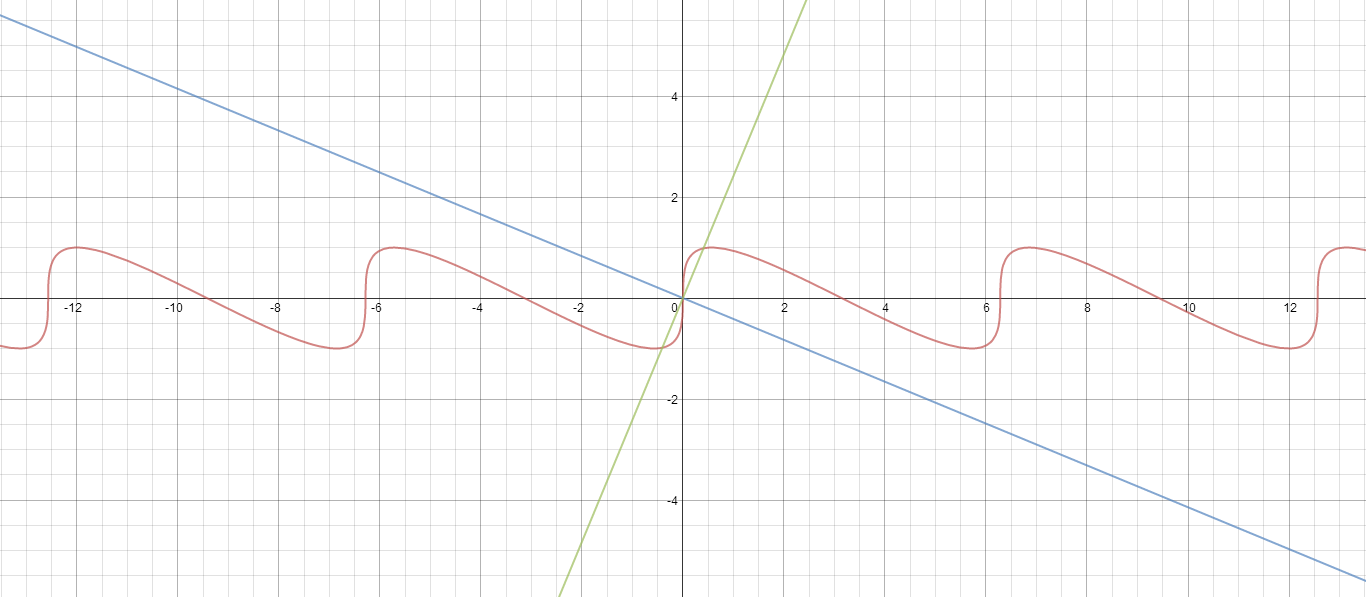# Perpendicular tangents

Calculus Level 3$(\alpha_1, \beta_1), \ (\alpha_2, \beta_2), \ \cdots \ , \ (\alpha_n, \beta_n)$ are the points on the curve $S \ : \ y=\sin (x+y) \ \ \forall x \in [-4\pi, \ 4\pi]$
such that the tangents at these points to $S$ are perpendicular to the line $l \ : \ (\sqrt {2}-1)x+y=0.$

Find the value of $\left\lfloor \displaystyle\sum_{k=1}^{n} |\alpha_k|-|\beta_k|\right\rfloor.$

Details and Assumptions

• $\lfloor{\cdots}\rfloor$ denotes the floor function (greatest integer function).

• Even though in the problem, a plural form of points is given, there may only be one such point that exists.

×

Problem Loading...

Note Loading...

Set Loading...